# 3D Shape Names

Here we will learn about 3D shapes names, including types of polyhedra (prisms, pyramids, platonic solids, irregular), non-polyhedra (cones, cylinders, spheres, hemispheres) and compound 3D shapes.

There are also 3D shapes names worksheets based on Edexcel, AQA and OCR exam questions which are printable, along with further guidance on where to go next if you’re still stuck.

## What are 3D shapes names?

3D shapes names are what solid shapes that have 3 dimensions (height, width and depth) are known as.

The properties of 3D shapes can help to classify them and recall their names.

To name a 3D shape it is useful to try and categorise it first.

### What are 3D shapes?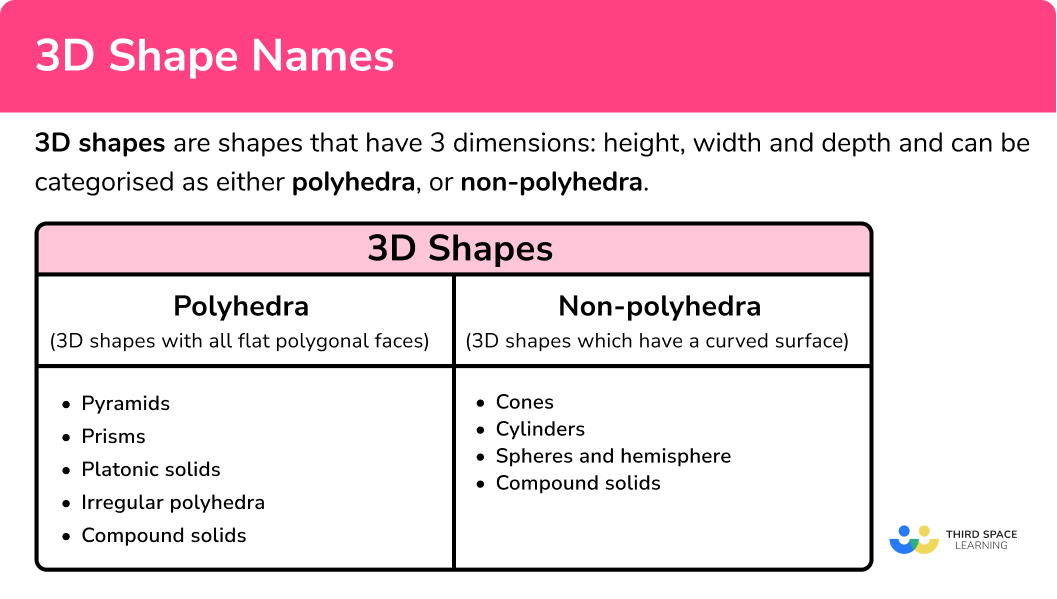### Polyhedra

Polyhedra are 3D shapes with flat faces, straight sides (edges) and sharp vertices.

All polyhedra can then be categorised as prisms, pyramids, platonic solids or irregular polyhedra.

Here are some examples of polyhedra.

*Some 3D shapes can fit into more than one category. For example, a tetrahedron is one of the platonic solids, but it is also a triangular based pyramid.

### Non-polyhedra

Non-polyhedra are 3D shapes that include curved surfaces. They may also have some flat faces, edges or vertices.

Here are some examples of non-polyhedra.

There are other categories of 3D shapes, but they are not needed for GCSE.

### Platonic solids

Some of the most famous polyhedra are called the Platonic solids named after the Greek philosopher and Mathematician, Plato. The platonic solids can also be referred to as regular polyhedra because their faces are regular polygons; each solid is made up of one type of regular polygon and can be inscribed inside a sphere.

In practical terms, this means that the solid can be used as a fair dice, where there is an equal chance of landing on each face.

For example,

### Prisms

A prism is a polyhedron that has congruent cross sections. In non-mathematical words, it is a 3D shape that can be sliced like a loaf of bread, and each slice will look like the same flat shape.

For example, the cuboid below has a rectangular cross section that is the same for the entire depth of the shape.

For example, the triangular prism below has a triangular cross section that is the same for the entire depth of the shape.

Exam questions involving prisms may ask you to calculate the volume, calculate the surface area, draw the net, or count the number of edges, faces and vertices.

### Cylinders

Geometrically a cylinder is like a prism. The cross sections of a cylinder are congruent circles.

### Face, edges and vertices

Faces, edges and vertices are words to describe different parts of 3D shapes.

The cube in the table above has \bf{6} faces, \bf{12} edges and \bf{8} vertices.

Step-by-step guide: Faces, edges and vertices

### Pyramids

A pyramid is a polyhedron that has a flat polygonal base and an apex. It has triangular side faces which slope to meet each other at the apex.

Regular pyramids

The base shape of a regular pyramid is a regular polygon (A 2D shape with straight equal sides).

All the lateral edges (edges leading from the base to the apex) are equal in length.

Irregular pyramids

The base shape of an irregular pyramid is an irregular polygon (a 2D shape with straight sides which vary in length).

The lateral edges may be different in length.

For all types of pyramids the cross sectional areas are similar to each other as illustrated in the diagrams below.

Right pyramids

If the apex of the pyramid lies directly on top of the centre of the base, the pyramid is a right pyramid.

Oblique pyramids

If the apex of the pyramid does not lie directly on top of the centre of the base, the pyramid is an oblique pyramid.

This is illustrated in the diagrams below. The cross sectional areas of both right pyramids and oblique pyramids are similar to each other.

For example,

Cones

Cones can be described like pyramids; they have a circular base shape and an apex. Their cross-sectional areas are similar circles. However, unlike pyramids, cones do not have sloping triangular side faces but instead they have a curved side surface.

Frustums

If the apex of a pyramid or cone is sliced off then the remaining shape is known as a frustum. The mathematical term for ‘slicing off an apex’ is ‘truncating’. So a frustum can also be described as a truncated pyramid or truncated cone.

### Irregular polyhedra

If a 3D shape is a polyhedron (it is made up of flat polygonal faces) but it cannot be categorised as a prism, pyramid or platonic solid, then it is an irregular polyhedron. An irregular polyhedron can have faces which are regular polygons or irregular polygons, or a combination of both.

For example, the 3D shape below is a well recognised irregular polyhedron. This solid is made up of regular pentagons and regular hexagons and is the design of a football.

### Compound solids

A compound solid is a 3D shape that is made up of other identifiable 3D shapes.

For example, this compound solid is a polyhedron and made up of a cuboid and a triangular prism.

This compound solid is a non-polyhedra and is made up of a cylinder and a cone.

## How to categorise and name a 3D shape

In order to categorise and name a 3D shape:

1. Identify if the 3D shape is:
i)  a polyhedron (all flat polygonal faces) – go to step 2.
ii) a non-polyhedron (includes a curved surface) – go to step 3.
2. Identify if all the faces are the same regular shape.
i) If yes, this is one of the Platonic solids (tetrahedron, cube, octahedron, dodecahedron or icosahedron).
ii) If no, continue to step 3.
3. Identify if the 3D shape is:
a) a pyramid or cone (3D shape with a base, an apex and similar cross-sectional areas).
b) a prism or cylinder (3D shape with congruent cross-sectional areas).
c) neither.
4. Name the shape.
a) For pyramids: the name of the base shape often forms the name of the 3D shape.
b) For prisms: the name of the cross-sectional area often forms the name of the 3D shape.
c) i) For neither (polyhedron): This is an irregular polyhedron or a compound solid.
ii) For neither (non-polyhedron): those needed to be named on the GCSE syllabus are spheres and hemispheres.

### Explain how to categorise and name a 3D shape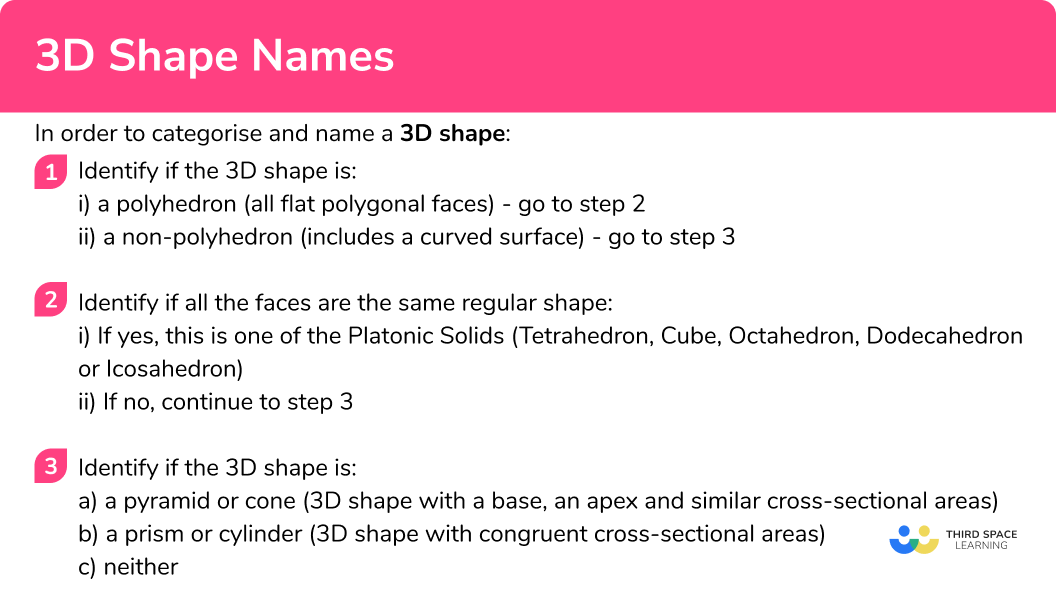### Explain how to categorise and name a 3D shape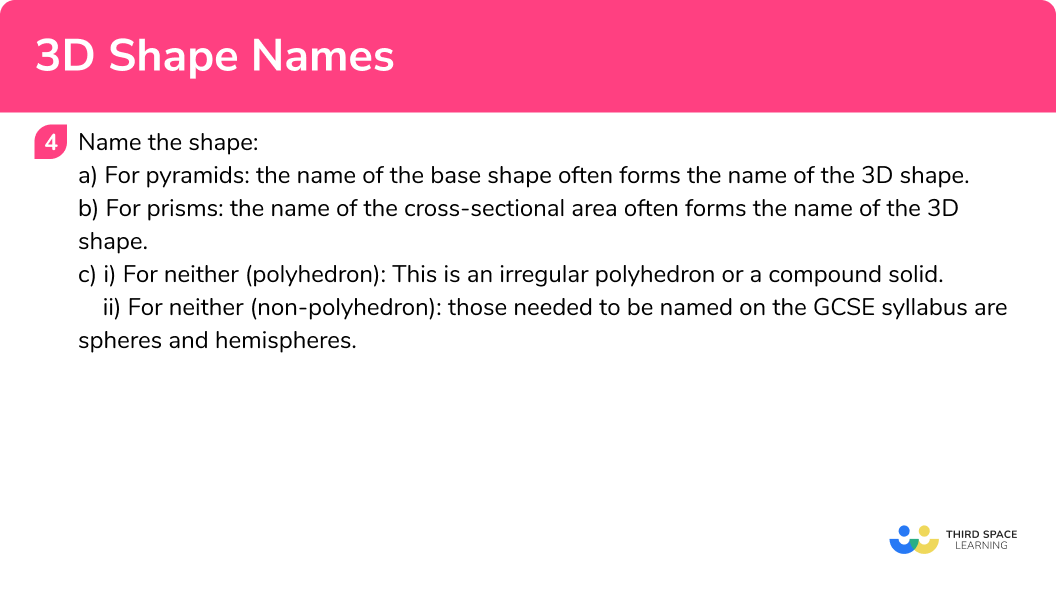### Related lessons on 3D shapes

3D shape names is part of our series of lessons to support revision on 3D shapes. You may find it helpful to start with the main 3D shapes lesson for a summary of what to expect, or use the step by step guides below for further detail on individual topics. Other lessons in this series include:

## 3D shapes names examples

### Example 1: naming a 3D shape from its net

What 3D shape can be formed from this net?

1. Identify if the 3D shape is:
i)  a polyhedron (all flat polygonal faces) – go to step 2.
ii) a non-polyhedron (includes a curved surface) – go to step 3.

All the 2D shapes that make up this net are polygons; they are all rectangles. The 3D shape it will form is therefore a polyhedron.

2Identify if all the faces are the same regular shape.
i) If yes, this is one of the Platonic solids (tetrahedron, cube, octahedron, dodecahedron or icosahedron).
ii) If no, continue to step 3.

The faces are not the same; they are different size rectangles.

This is not a platonic solid.

3Identify if the 3D shape is:
a) a pyramid or cone (3D shape with a base, an apex and similar cross-sectional areas).
b) a prism or cylinder (3D shape with congruent cross-sectional areas).
c) neither.

Imagine the net folding up into a 3D shape.

The cross-sectional areas are congruent as shown by these yellow rectangles. This 3D shape is a type of prism.

4Name the shape.
a) For pyramids: the name of the base shape often forms the name of the 3D shape.
b) For prisms: the name of the cross-sectional area often forms the name of the 3D shape.
c) i) For neither (polyhedron): This is an irregular polyhedron or a compound solid***.
ii) For neither (non-polyhedron): those needed to be named on the GCSE syllabus are spheres and hemispheres.

This is a rectangular prism which should be referred to as a cuboid.

### Example 2: naming a 3D shape from a 3D image and description

The following 3D shape is made up of 4 equilateral triangles. What is the name of this 3D shape?

Identify if the 3D shape is:
i)  a polyhedron (all flat polygonal faces) – go to step 2.
ii) a non-polyhedron (includes a curved surface) – go to step 3.

Identify if all the faces are the same regular shape.
i) If yes, this is one of the Platonic solids (tetrahedron, cube, octahedron, dodecahedron or icosahedron).
ii) If no, continue to step 3.

### Example 3: naming a 3D shape from a 3D image

What is the name of this 3D shape?

Identify if the 3D shape is:
i) a polyhedron (all flat polygonal faces) – go to step 2.
ii) a non-polyhedron (includes a curved surface) – go to step 3.

Identify if all the faces are the same regular shape.
i) If yes, this is one of the Platonic solids (tetrahedron, cube, octahedron, dodecahedron or icosahedron).
ii) If no, continue to step 3.

Identify if the 3D shape is:
a) a pyramid or cone (3D shape with a base, an apex and similar cross-sectional areas).
b) a prism or cylinder (3D shape with congruent cross-sectional areas).
c) neither.

Name the shape.
a) For pyramids: the name of the base shape often forms the name of the 3D shape.
b) For prisms: the name of the cross-sectional area often forms the name of the 3D shape.
c)
i) For neither (polyhedron): This is an irregular polyhedron or a compound solid.
ii) For neither (non-polyhedron): those needed to be named on the GCSE syllabus are spheres and hemispheres.

### Example 4: naming a 3D shape from a 3D image and description

The following 3D shape is made up of 8 equilateral triangles. What is the name of this 3D shape?

Identify if the 3D shape is:
i)  a polyhedron (all flat polygonal faces) – go to step 2.
ii) a non-polyhedron (includes a curved surface) – go to step 3.

Identify if all the faces are the same regular shape.
i) If yes, this is one of the Platonic solids (tetrahedron, cube, octahedron, dodecahedron or icosahedron).
ii) If no, continue to step 3.

### Example 5: naming a 3D shape from its net

What 3D shape can be formed from this net?

Identify if the 3D shape is:
i)  a polyhedron (all flat polygonal faces) – go to step 2.
ii) a non-polyhedron (includes a curved surface) – go to step 3.

Identify if the 3D shape is:
a) a pyramid or cone (3D shape with a base, an apex and similar cross-sectional areas).
b) a prism or cylinder (3D shape with congruent cross-sectional areas).
c) neither.

Name the shape.
a) For pyramids: the name of the base shape often forms the name of the 3D shape.
b) For prisms: the name of the cross-sectional area often forms the name of the 3D shape.
c)
i) For neither (polyhedron): This is an irregular polyhedron or a compound solid.
ii) For neither (non-polyhedron): those needed to be named on the GCSE syllabus are spheres and hemispheres.

### Example 6: categorising and describing a 3D shape

Below is an image of a compound solid.

(a) Is this solid a type of pyramid or prism?

(b) What 3D shapes make up this compound solid?

Identify if the 3D shape is:
i)  a polyhedron (all flat polygonal faces) – go to step 2.
ii) a non-polyhedron (includes a curved surface) – go to step 3.

Identify if all the faces are the same regular shape.
i) If yes, this is one of the Platonic solids (tetrahedron, cube, octahedron, dodecahedron or icosahedron).
ii) If no, continue to step 3.

Identify if the 3D shape is:
a) a pyramid or cone(3D shape with a base, an apex and similar cross-sectional areas).
b) a prism or cylinder (3D shape with congruent cross-sectional areas).
c) neither
.

Name the shape.
a) For pyramids: the name of the base shape often forms the name of the 3D shape.
b) For prisms: the name of the cross-sectional area often forms the name of the 3D shape.
c)
i) For neither (polyhedron): This is an irregular polyhedron or a compound solid.
ii) For neither (non-polyhedron): those needed to be named on the GCSE syllabus are spheres and hemispheres.

### Common misconceptions

• Mixing up 3D and 2D shape names

Make sure you know the correct 3D shape names and avoid common mistakes involving 2D shape names like the following examples:

Cube – common incorrect name ‘3D square’

Cuboid – common incorrect name ‘3D rectangle’

Tetrahedron – common incorrect name ‘3D triangle’

• Using a descriptive 3D shape name instead of the correct mathematical name

Some 3D shapes have descriptive names or can be likened to certain categories of 3D shapes but must be referred to by the correct mathematical name. Here is a list of examples which are part of the GCSE syllabus.

Circular prism = cylinder

Rectangular prism = cuboid

Circular based pyramid = cone

Triangular based pyramid (equilateral triangles) = tetrahedron

Ball = sphere

Half a ball = hemisphere

### Practice 3D shape names questions

1. Below is the net of a 3D shape.What is the name of the 3D shape that can be formed from this net?

3D squareCubeCuboidAll the faces are the same regular shape; squares. Therefore this net will form one of the five platonic solids. A cube is made up of 6 squares.

2. Below is an image of a 3D shape.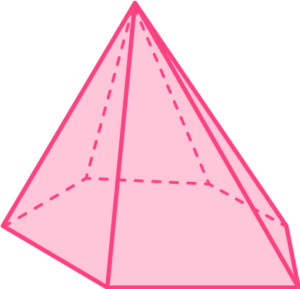What is the name of this 3D shape?

HexagonHexagonal pyramidHexahedronHexagonal prismThis 3D shape has a base, an apex and similar cross-sectional areas: it is therefore a pyramid. The base shape is a hexagon, hence, it is a hexagonal pyramid.

3. Below is an image of a 3D shape.What is the name of this 3D shape?

Right pyramidTriangular pyramidTetrahedronTriangular prismThis 3D shape has congruent cross-sectional areas: it is therefore a prism. The shape of the cross-section is a triangle, hence, it is a triangular prism.

4. Below is an image of a 3D shape which is made up of 12 regular pentagons.What is the name of this 3D shape?

DodecahedronPentagonal prismPentahedronIcosahedronAll the faces are the same regular shape; regular pentagons. Therefore this 3D shape is one of the five platonic solids. A dodecahedron is made up of 12 regular pentagons.

5. Below is the net of a 3D shape.What is the name of the 3D shape that can be formed from this net?

Irregular triangleRegular TetrahedronTriangular based pyramidTriangular prismThe triangle in the middle of the net will be the base and the other triangles will fold up and meet at an apex.

6. Below is an image of a 3D shape.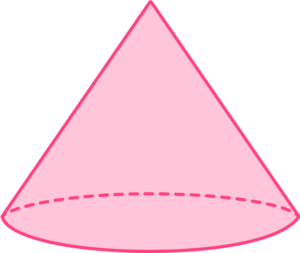What is the name of this 3D shape?

CylinderCircular based pyramidSphereConeThis 3D shape has a base, an apex and similar cross-sectional areas, it is therefore a pyramid or cone.  A cone can look ‘pyramid-like’ with a circular base.

### 3D shapes names GCSE questions

1. Here are five images of 3D shapes. From the list provided choose the best mathematical term to describe each image.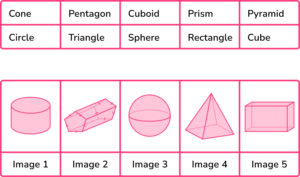(3 marks)

Image 1 = Cylinder

Image 2 = Prism

Image 3 = Sphere

Image 4 = Pyramid

Image 5 = Cuboid

(1)

(1)

(1)

2. Write down the mathematical name for the 3D shapes being described below.

(a) A polyhedron formed of six squares inscribed inside a sphere.

(b) A non-polyhedron formed of two circles and one rectangle which curves round to create a curved surface.

(c) A polyhedron formed of one pentagon and five triangles which meet at an apex.

(3 marks)

(a) Cube

(1)

(b) Cylinder

(1)

(c) Pentagonal based pyramid

(1)

3. (a) What 3D shape can be formed from this net?(b) What two 3D shapes make up the compound solid depicted in the image below?(3 marks)

(a) Triangular prism

(1)

(b) Cube

(1)

Square based pyramid

(1)

## Learning checklist

You have now learned how to:

• Identify 3D shapes from 2D representations, nets or written properties

## Still stuck?

Prepare your KS4 students for maths GCSEs success with Third Space Learning. Weekly online one to one GCSE maths revision lessons delivered by expert maths tutors.

Find out more about our GCSE maths tuition programme.